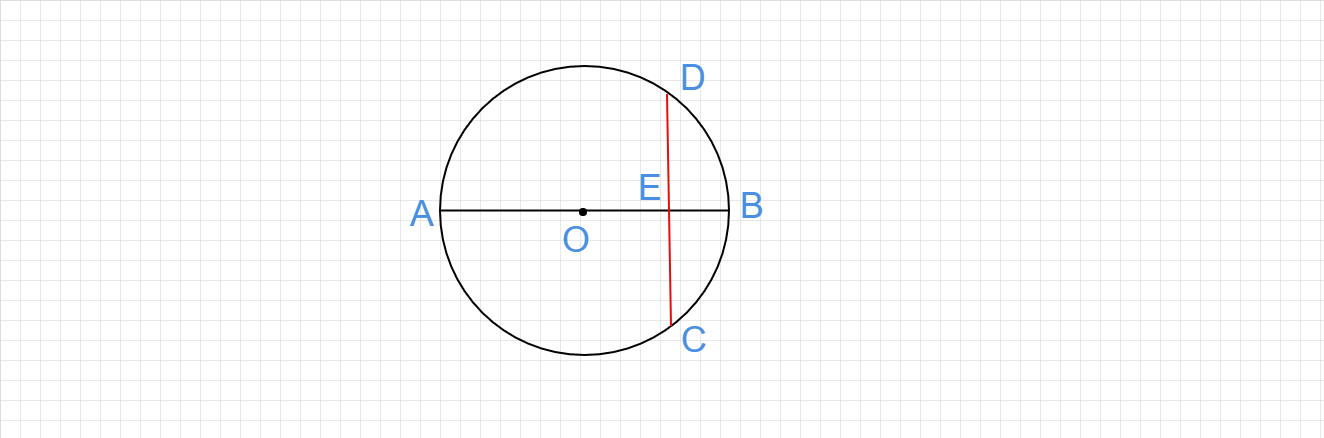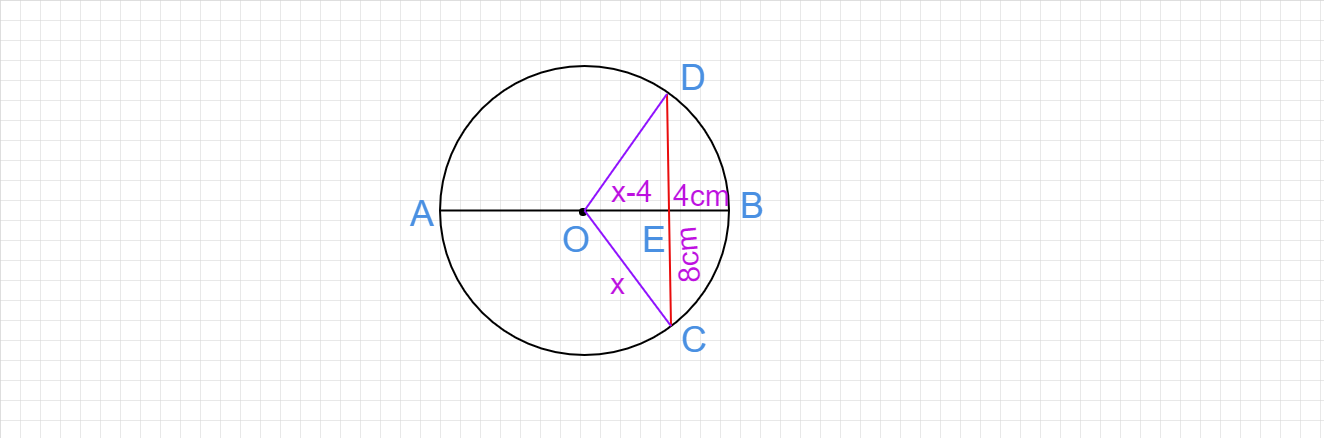"
">

# In the given figure, a circle with center O is given in which a diameter AB bisects the chord CD at a point E such that $CE=E D=8 cm$ and $E B=4 cm$. Find the radius of the circle."

Given :

In the given circle, O is the center, and diameter AB bisects the chord CD at the point E.

$CE=E D=8 cm$ and $E B=4 cm$.

To do :

We have to find the radius of the circle.

Solution :

Let the radius of the circle be 'x'.From the figure, $OB = x$

$OB = OE+EB$

$OE = OB-EB$

$OE = x-4$

We know that "the perpendicular bisector of the chord passes through the center of the circle".

So, OEC is a right-angled triangle.

$x^2 = (x-4)^2+8^2$

$x^2 = x^2 -8x + 16+64$

$x^2=x^2-8x+80$

$x^2-x^2+8x=80$

$8x = 80$

$x = \frac{80}{8}$

$x = 10$.

Therefore, the radius of the circle is 10 cm.

Updated on: 10-Oct-2022

27 Views